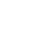17.1.2 Gibbs Free Energy & the Equilibrium Constant

Gibbs Free Energy & the Equilbrium Constant

Gibbs Free Energy & the Equilibrium Constant

• The equilibrium constant, Kc, gives no information about the individual rates of reaction
• It is independent of the kinetics of the reaction
• The equilibrium constant, Kc, is directly related to the Gibbs free energy change, ΔG, according to the following (Van’t Hoff’s) equation:

ΔG = –RT lnK

• ΔG = Gibbs free energy change (kJ mol1)
• R = gas constant (8.31 J K-1 mol-1)
• T = temperature (Kelvin, K)
• K = equilibrium constant
• This equation is provided in section 1 of the data booklet

Exam Tip

When completing calculations using the ΔG = –RT lnK equation, you have to be aware that:

• ΔG is measure in kJ mol1
• R is measured in J K-1 mol-1

This means that one of these values will need adjusting by a factor of 1000

• This relationship between the equilibrium constant, Kc, and Gibbs free energy change, ΔG, can be used to determine whether the forward or backward reaction is favoured

The relationship between the equilibrium constant, Kc, and Gibbs free energy change, ΔG

• At a given temperature, a negative ΔG value for a reaction indicates that:
• The reaction is feasible / spontaneous
• The equilibrium concentration of the products is greater than the equilibrium concentration of the reactants
• The value of the equilibrium constant is greater than 1
• As ΔG becomes more negative:
• The forward reaction is favoured more
• The value of the equilibrium constant increases

Free Energy & Equilibrium Calculations

• The relationship between Gibbs free energy change, ΔG, temperature and the equilibrium constant, Kc, is described by the equation:

ΔG = –RT lnK

• The rearrangement of this equation makes it possible to:
• Calculate the equilibrium constant
• Deduce the position of equilibrium for the reaction

lnK = –CloseClose